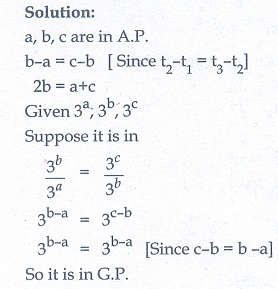Home | | Maths 10th Std | Exercise 2.7: Geometric Progression

# Exercise 2.7: Geometric Progression

Maths Book back answers and solution for Exercise questions - Mathematics : Numbers and Sequences: Geometric Progression: Exercise Questions with Answer

### Exercise 2.7

1. Which of the following sequences are in G.P.?

(i) 3,9,27,81,…

(ii) 4,44,444,4444,...

(iii) 0.5,0.05,0.005,…

(iv) 1/3 , 1/6 , 1/12 ,...

(v) 1, − 5, 25, −125,…

(vi) 120,60,30,18,…

(vii)16, 4, 1, 1/4 , ...2. Write the first three terms of the G.P. whose first term and the common ratio are given below.

(i) a = 6, r = 3

(ii) a = √2, r =  √2

(iii) a = 1000, r = 2/53. In a G.P. 729, 243, 81,… find t7.4. Find x so that x + 6, x + 12 and x + 15 are consecutive terms of a Geometric Progression.5. Find the number of terms in the following G.P.

(i) 4,8,16,…,8192?

(ii)6. In a G.P. the 9th term is 32805 and 6th term is 1215. Find the 12th term.7. Find the 10th term of a G.P. whose 8th term is 768 and the common ratio is 2.8. If a, b, c are in A.P. then show that 3a , 3b , 3c   are in G.P.9. In a G.P. the product of three consecutive terms is 27 and the sum of the product of two terms taken at a time is 57/2 . Find the three terms.10. A man joined a company as Assistant Manager. The company gave him a starting salary of ₹60,000 and agreed to increase his salary 5% annually. What will be his salary after 5 years?11. Sivamani is attending an interview for a job and the company gave two offers to him. Offer A: ₹20,000 to start with followed by a guaranteed annual increase of 6% for the first 5 years. Offer B: ₹22,000 to start with followed by a guaranteed annual increase of 3% for the first 5 years.

What is his salary in the 4th  year with respect to the offers A and B?12. If abc are three consecutive terms of an A.P. and xyz are three consecutive terms of a G.P. then prove that xb −c × yc −a × za −b = 1.1.(i) G.P (ii) not a G.P (iii) G.P (iv) G.P (v) G.P (vi) not a G.P (vii) G.P

2.(i) 6,18,54 (ii) √2 ,2, 2√2 (iii) 1000,400,160

3. 1

4. -18

5.(i) 12 (ii) 7

6. 5 × (311)

7. 3072

9. 9/2, 3, 2

10. ₹ 76577

11. ₹ 23820, ₹ 24040

Tags : Problem Questions with Answer, Solution | Mathematics , 10th Mathematics : UNIT 2 : Numbers and Sequences
Study Material, Lecturing Notes, Assignment, Reference, Wiki description explanation, brief detail
10th Mathematics : UNIT 2 : Numbers and Sequences : Exercise 2.7: Geometric Progression | Problem Questions with Answer, Solution | Mathematics